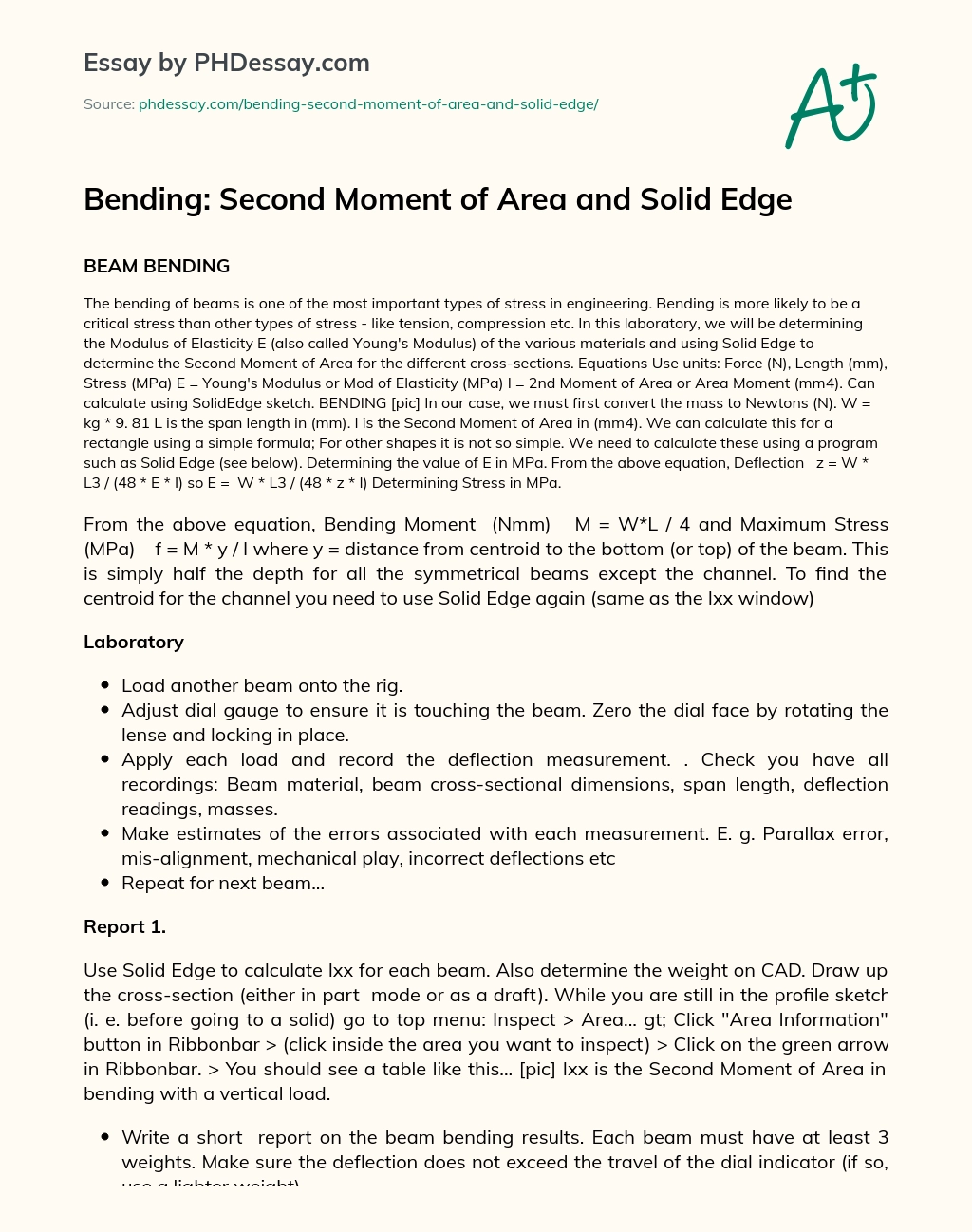Last Updated 07 Sep 2020

# Bending: Second Moment of Area and Solid Edge

Category Physics
Words 580 (3 pages)
Views 558

## BEAM BENDING

The bending of beams is one of the most important types of stress in engineering. Bending is more likely to be a critical stress than other types of stress - like tension, compression etc. In this laboratory, we will be determining the Modulus of Elasticity E (also called Young's Modulus) of the various materials and using Solid Edge to determine the Second Moment of Area for the different cross-sections.

Equations Use units: Force (N), Length (mm), Stress (MPa) E = Young's Modulus or Mod of Elasticity (MPa) I = 2nd Moment of Area or Area Moment (mm4). Can calculate using SolidEdge sketch. BENDING [pic] In our case, we must first convert the mass to Newtons (N). W = kg * 9. 81 L is the p length in (mm). I is the Second Moment of Area in (mm4). We can calculate this for a rectangle using a simple formula; For other shapes it is not so simple. We need to calculate these using a program such as Solid Edge (see below). Determining the value of E in MPa. From the above equation, Deflection   z = W * L3 / (48 * E * I) so E =  W * L3 / (48 * z * I) Determining Stress in MPa.

Order custom essay Bending: Second Moment of Area and Solid Edge with free plagiarism report

GET ORIGINAL PAPER

From the above equation, Bending Moment  (Nmm)   M = W*L / 4 and Maximum Stress (MPa)    f = M * y / I where y = distance from centroid to the bottom (or top) of the beam. This is simply half the depth for all the symmetrical beams except the channel. To find the centroid for the channel you need to use Solid Edge again (same as the Ixx window)

## Laboratory

• Load another beam onto the rig.
• Adjust dial gauge to ensure it is touching the beam. Zero the dial face by rotating the lense and locking in place.
• Apply each load and record the deflection measurement. . Check you have all recordings: Beam material, beam cross-sectional dimensions, p length, deflection readings, masses.
• Make estimates of the errors associated with each measurement. E. g. Parallax error, mis-alignment, mechanical play, incorrect deflections etc
• Repeat for next beam...

### Report 1.

Use Solid Edge to calculate Ixx for each beam. Also determine the weight on CAD. Draw up the cross-section (either in part  mode or as a draft). While you are still in the profile sketch (i. e. before going to a solid) go to top menu: Inspect > Area... gt; Click "Area Information" button in Ribbonbar > (click inside the area you want to inspect) > Click on the green arrow in Ribbonbar. > You should see a table like this... [pic] Ixx is the Second Moment of Area in bending with a vertical load.

• Write a short  report on the beam bending results. Each beam must have at least 3 weights. Make sure the deflection does not exceed the travel of the dial indicator (if so, use a lighter weight).
• Using the equations above, calculate the value of E. Compare these values to the values obtained from the internet.
• E. g. Matweb. Show the working for 1 example calculation, but only give the rest of the answers in a table. Use Excel to do your calculations.
• Determine the maximum stress  for each mass (load) added to the beams.

Discuss any sources of error in the experiment - esp measurements - and how they might affect the results. Specify an overall error for your calculation of E.  Using the dial gauge to measure deflection in the beam while under a load of 500g. [pic] [pic] The face of the dial gauge can be rotated to zero the scale.This essay was written by a fellow student. You can use it as an example when writing your own essay or use it as a source, but you need cite it.

## Get professional help and free up your time for more important courses

Starting from 3 hours delivery 450+ experts on 30 subjects
get essay help 124  experts online

Did you know that we have over 70,000 essays on 3,000 topics in our database?

Explore how the human body functions as one unit in harmony in order to life

Bending: Second Moment of Area and Solid Edge. (2016, Nov 21). Retrieved from https://phdessay.com/bending-second-moment-of-area-and-solid-edge/